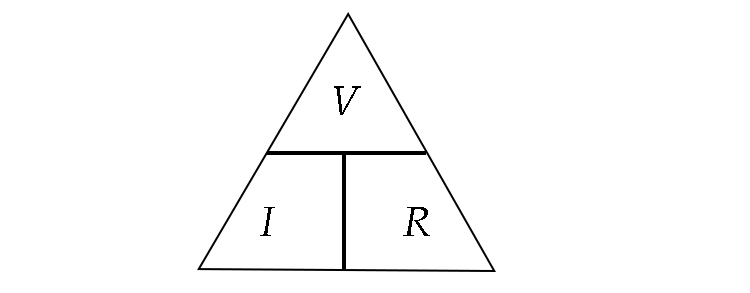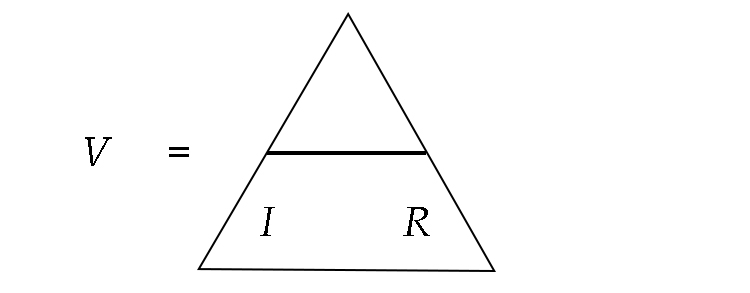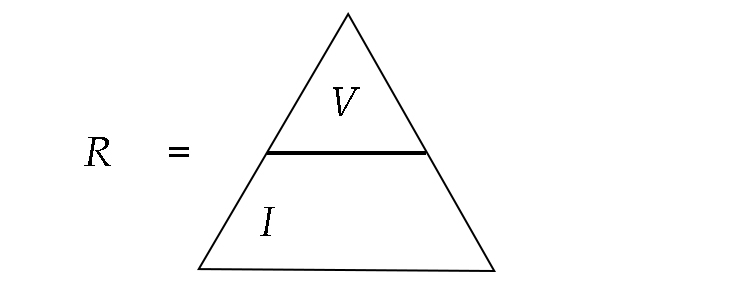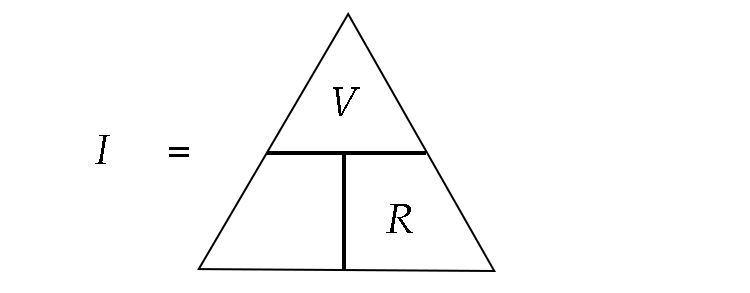# Alternative method to remember Ohm's law

Another way of remembering Ohm's law is to use a power triangle and remember Vault into an Indian Restaurant.

(See previous page)1. If you want to know what volts are, grab the V from the triangle and what are you left with is:Therefore:

V=IxxR

2. If you want to know what resistance is, grab the R from the triangle and what are you left with is:Therefore:

R=V/I

3. If you want to know what the amps are, grab the I from the triangle and see what you are left with:Therefore:

I=V/R